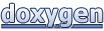# Assimp: aiMatrix3x3t< TReal > Class Template Reference

#### assimp - Open Asset Import Library

 Assimp  v3.1.1 (June 2014)
aiMatrix3x3t< TReal > Class Template Reference

Represents a row-major 3x3 matrix. More...

## Public Member Functions

aiMatrix3x3t ()

aiMatrix3x3t (TReal _a1, TReal _a2, TReal _a3, TReal _b1, TReal _b2, TReal _b3, TReal _c1, TReal _c2, TReal _c3)

aiMatrix3x3t (const aiMatrix4x4t< TReal > &pMatrix)
Construction from a 4x4 matrix. More...

TReal Determinant () const

bool Equal (const aiMatrix4x4t< TReal > &m, TReal epsilon=1e-6) const

aiMatrix3x3tInverse ()
Invert the matrix. More...

template<typename TOther >
operator aiMatrix3x3t< TOther > () const

bool operator!= (const aiMatrix4x4t< TReal > &m) const

aiMatrix3x3t operator* (const aiMatrix3x3t &m) const

aiMatrix3x3toperator*= (const aiMatrix3x3t &m)

bool operator== (const aiMatrix4x4t< TReal > &m) const

TReal * operator[] (unsigned int p_iIndex)

const TReal * operator[] (unsigned int p_iIndex) const

aiMatrix3x3tTranspose ()
Transpose the matrix. More...

## Static Public Member Functions

static aiMatrix3x3tFromToMatrix (const aiVector3t< TReal > &from, const aiVector3t< TReal > &to, aiMatrix3x3t &out)
A function for creating a rotation matrix that rotates a vector called "from" into another vector called "to". More...

static aiMatrix3x3tRotation (TReal a, const aiVector3t< TReal > &axis, aiMatrix3x3t &out)
Returns a rotation matrix for a rotation around an arbitrary axis. More...

static aiMatrix3x3tRotationZ (TReal a, aiMatrix3x3t &out)
Returns a rotation matrix for a rotation around z. More...

static aiMatrix3x3tTranslation (const aiVector2t< TReal > &v, aiMatrix3x3t &out)
Returns a translation matrix. More...

## Public Attributes

union {
struct {
TReal   a1

TReal   a2

TReal   a3

TReal   b1

TReal   b2

TReal   b3

TReal   c1

TReal   c2

TReal   c3

}

TReal   m 

TReal   mData 

};

## Detailed Description

### template<typename TReal> class aiMatrix3x3t< TReal >

Represents a row-major 3x3 matrix.

There's much confusion about matrix layouts (column vs. row order). This is always a row-major matrix. Not even with the aiProcess_ConvertToLeftHanded flag, which absolutely does not affect matrix order - it just affects the handedness of the coordinate system defined thereby.

## Constructor & Destructor Documentation

template<typename TReal >
 aiMatrix3x3t< TReal >::aiMatrix3x3t ( )
inline
template<typename TReal >
 aiMatrix3x3t< TReal >::aiMatrix3x3t ( TReal _a1, TReal _a2, TReal _a3, TReal _b1, TReal _b2, TReal _b3, TReal _c1, TReal _c2, TReal _c3 )
inline
template<typename TReal >
 aiMatrix3x3t< TReal >::aiMatrix3x3t ( const aiMatrix4x4t< TReal > & pMatrix )
inlineexplicit

Construction from a 4x4 matrix.

The remaining parts of the matrix are ignored.

## Member Function Documentation

template<typename TReal >
 TReal aiMatrix3x3t< TReal >::Determinant ( ) const
inline
template<typename TReal >
 bool aiMatrix3x3t< TReal >::Equal ( const aiMatrix4x4t< TReal > & m, TReal epsilon = `1e-6` ) const
inline
template<typename TReal >
 aiMatrix3x3t< TReal > & aiMatrix3x3t< TReal >::FromToMatrix ( const aiVector3t< TReal > & from, const aiVector3t< TReal > & to, aiMatrix3x3t< TReal > & out )
inlinestatic

A function for creating a rotation matrix that rotates a vector called "from" into another vector called "to".

Input : from, to which both must be normalized non-zero vectors Output: mtx – a 3x3 matrix in colum-major form Authors: Tomas M�ller, John Hughes "Efficiently Building a Matrix to Rotate One Vector to Another" Journal of Graphics Tools, 4(4):1-4, 1999

template<typename TReal >
 aiMatrix3x3t< TReal > & aiMatrix3x3t< TReal >::Inverse ( )
inline

Invert the matrix.

If the matrix is not invertible all elements are set to qnan. Beware, use (f != f) to check whether a TReal f is qnan.

template<typename TReal >
template<typename TOther >
 aiMatrix3x3t< TReal >::operator aiMatrix3x3t< TOther > ( ) const
template<typename TReal >
 bool aiMatrix3x3t< TReal >::operator!= ( const aiMatrix4x4t< TReal > & m ) const
inline
template<typename TReal >
 aiMatrix3x3t< TReal > aiMatrix3x3t< TReal >::operator* ( const aiMatrix3x3t< TReal > & m ) const
inline
template<typename TReal >
 aiMatrix3x3t< TReal > & aiMatrix3x3t< TReal >::operator*= ( const aiMatrix3x3t< TReal > & m )
inline
template<typename TReal >
 bool aiMatrix3x3t< TReal >::operator== ( const aiMatrix4x4t< TReal > & m ) const
inline
template<typename TReal >
 TReal * aiMatrix3x3t< TReal >::operator[] ( unsigned int p_iIndex )
inline
template<typename TReal >
 const TReal * aiMatrix3x3t< TReal >::operator[] ( unsigned int p_iIndex ) const
inline
template<typename TReal >
 aiMatrix3x3t< TReal > & aiMatrix3x3t< TReal >::Rotation ( TReal a, const aiVector3t< TReal > & axis, aiMatrix3x3t< TReal > & out )
inlinestatic

Returns a rotation matrix for a rotation around an arbitrary axis.

Parameters
 a Rotation angle, in radians axis Axis to rotate around out To be filled
template<typename TReal >
 aiMatrix3x3t< TReal > & aiMatrix3x3t< TReal >::RotationZ ( TReal a, aiMatrix3x3t< TReal > & out )
inlinestatic

Returns a rotation matrix for a rotation around z.

Parameters
Returns
Reference to the output matrix
template<typename TReal >
 aiMatrix3x3t< TReal > & aiMatrix3x3t< TReal >::Translation ( const aiVector2t< TReal > & v, aiMatrix3x3t< TReal > & out )
inlinestatic

Returns a translation matrix.

Parameters
 v Translation vector out Receives the output matrix
Returns
Reference to the output matrix
template<typename TReal >
 aiMatrix3x3t< TReal > & aiMatrix3x3t< TReal >::Transpose ( )
inline

Transpose the matrix.

## Member Data Documentation

 union { ... }
template<typename TReal >
 TReal aiMatrix3x3t< TReal >::a1
template<typename TReal >
 TReal aiMatrix3x3t< TReal >::a2
template<typename TReal >
 TReal aiMatrix3x3t< TReal >::a3
template<typename TReal >
 TReal aiMatrix3x3t< TReal >::b1
template<typename TReal >
 TReal aiMatrix3x3t< TReal >::b2
template<typename TReal >
 TReal aiMatrix3x3t< TReal >::b3
template<typename TReal >
 TReal aiMatrix3x3t< TReal >::c1
template<typename TReal >
 TReal aiMatrix3x3t< TReal >::c2
template<typename TReal >
 TReal aiMatrix3x3t< TReal >::c3
template<typename TReal >
 TReal aiMatrix3x3t< TReal >::m
template<typename TReal >
 TReal aiMatrix3x3t< TReal >::mData
The documentation for this class was generated from the following files:
Generated on Sun Feb 21 2016 19:42:29 for Assimp by1.8.11